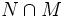# Normal and self-centralizing implies normality-large

This article gives the statement and possibly, proof, of an implication relation between two subgroup properties. That is, it states that every subgroup satisfying the first subgroup property (i.e., self-centralizing normal subgroup) must also satisfy the second subgroup property (i.e., normality-large normal subgroup)
View all subgroup property implications | View all subgroup property non-implications
Get more facts about self-centralizing normal subgroup|Get more facts about normality-large normal subgroup

## Statement

Any Normal subgroup (?) of a group that is self-centralizing, is also normality-large: its intersection with every nontrivial normal subgroup is nontrivial.

## Proof

Given: A group$G$, a normal subgroup$N$, such that$C_G(N) \le N$.

To prove: If$M$ is a nontrivial normal subgroup of$G$ such that$M \cap N$ is trivial, then$M$ is trivial.

Proof: Consider the commutator$[N,M]$. This is contained both in$N$ and in$M$, since they are both normal. Hence it is contained in$N \cap M$, which is trivial. Thus, every element of$N$ commutes with every element of$M$, so$M \le C_G(N)$. Since$N$ is self-centralizing, we get$M \le N$. Since$N \cap M$ is trivial, we get that$M$ is trivial.